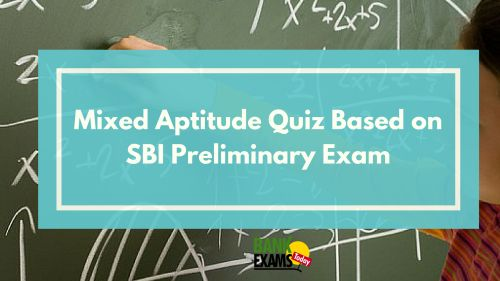New Students Offer - Use Code HELLO

# Mixed Aptitude Quiz Based On SBI Preliminary Exam#### Ques 1.

Bus starts running at the speed of 50 kmph .The speed of the bus increases by 3 kmph at end of every one hour .What will be the distance covered at the end of 7 hours from the start of the journey ?

Solution :

Total Distance = n/2 ( 2a + (n-1)d)

= 7/2 (2*50+(7-1)*3)
= 413 Km

#### Ques 2.

What will be the difference between the Compound Interest and Simple Interest earned on a sum of 2000 at the rate of 12 pcpa at end of 2 years ?

Solution :

Difference for 2 years = (p* r^2)/(100)^2

= 2000*12^2/100^2
=2000*144/10000
=28.8

#### Ques 3.

50       25       37.5      93.75       328.125      ?

Solution :

50*0.5=25
25*1.5=37.5
37.5*2.5=93.75
93.75*3.5=328.125
328.125*4.5=1476.5625

#### Ques 4.

Siva started a business investing 120000 . After 6 months Karan joined with 180000. After another 6 moths Giri also joined them with 2.7lakh . The profit earned at the end of 3 years when Siva started the business should be distributed among 3 persons in the ratio of ?

Solution :

Ratio = (120000 * 36 months ) : ( 180000 * 30months ) : (270000*24months)
= 4:5:6

#### Ques 5.

The cost of 26 CPUs and 6 Monitors is 13800 and the cost of 13 CPUs and 7 Monitors is 14200. What is the cost of 10 Monitors ?

Solution :

26C + 6M = 13800 ---(i)
13C + 7M =  14200 ---(ii)

From i & ii

M = 1825
10 Monitors = 1825*10=18250

#### Ques 6.

Hari has some hens and some cows . The total number of animals heads is 40 and total number of legs is 112 .How many hens does Hari have ?

Solution :

Assume all animals are hens , hence total legs = 40*2=80

Assume all animals are cows , hence total legs = 40*4=160

From question total legs =112

80.                        160

112

48                           32

= 48:32=3:2

Hens = 3/5  * 40 = 24

#### Ques 7.

A bag contains 8 black , 7 green , 6 yellow balls . What is the probability that 3 balls drawn at random are either black or green ?

Solution :

= {(8C3 + 7C3 )/(21C3) }= 13/190

#### Ques 8.

If edge of cube is increased by 50% then by what percentage will have the volume of cube increases ?

Solution :

Volume =100
New Volume = 100 *1.5*1.5*1.5 =337.5

Increased volume = 337.5-100=237.5

#### Ques 9.

Find Approximate value :   ? *5*4.9  - 13.11*4.01*4 = 117

Solution :

25x = 117+13*16
25x = 117+208
X = 325/25=13

#### Ques 10.

2x^2 + 11x +15=0 ; 4y^2 + 22y+24=0 , Find relation between x and y ?

Solution :

2x^2+6x+5x+15 = 0
==> x = -6/2 ; -5/2

4y^2 +16y+6y+ 24 =0
==> y = -16/4 ; -6/4 = -4; -3/2

Hence no relationship can be established.